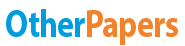# Jetblue - the Dcf Valuation Method

Essay by   •  May 3, 2017  •  Case Study  •  777 Words (4 Pages)  •  701 Views

## Essay Preview: Jetblue - the Dcf Valuation Method

Report this essay
Page 1 of 4

2. The DCF Valuation Method

DCF valuation approach calculates the worth of JetBlue based on the present value of the firm’s future cash flow. We use the estimated date given in the Exhibit 13 to forecast the free cash flows from 2002 to 2010. The key assumption of the forecast given in the Exhibit 13 is that the revenue, depreciation and capital expenditure increase with the number of the aircraft and the expected inflation. After 2010, we assume the revenue will grow at a constant rate and the cash flow growth will stabilize after 2011. In the long term, the growth rate of the company will approach the long -term US GDP nominal growth rate. Therefore, we estimate the growth rate at 4.68%. (see Exhibt2)

We use the CAMP model to determine the cost of equity of JetBlue, which equals to 11.03%. We use the proxy company Southwest’s D/E ratio and cost of debt as JetBlue’s estimated cost of debt to calculate the cost of capital, which equals to 10.40%. Therefore, the firm value of JetBlue equals \$1891.33 million, and the equity value and share price equals \$1516.90 million and \$43.22, respectively. Calculation is shown in Exhibit 1 to Exhibit 3.

Exhibit 1 Estimate WACC of JetBlue

Without required data of JetBlue, we use the information of Southwest Airlines. As the pioneer and dominant company in US’s low-fare airlines, Southwest can be a comparable company for JetBlue. Thus, the estimation about WACC of Southwest is a reasonable proxy for JetBlue's because they have similar business structure and similar systematic risk.

Cost of equity

We use the levered beta of Southwest to estimate the levered beta JetBlue. We assume that JetBlue has the same D/E ratio with Southwest. As Southwest has 776.8 million shares outstanding and the share price is \$20.69 currently, the total equity is \$16071.99 million. With \$1842 million debt, the D/E ratio equals 0.11. Therefore, the beta of JetBlue can be calculated as followed.

[pic 1]

[pic 2]

Given that the risk-free rate and the market risk premium equal 5% and 5.5% respectively, we can calculate the cost of equity according to the CAPM model.

[pic 3]

Cost of debt

We use the yield to maturity of long-term maturity debt of Southwest to estimate the cost of debt of JetBlue, which is 7.41%

WACC

[pic 4]

Exhibit 2 free cash flow of JetBlue

We have used the following assumptions supplied by the JetBlue management forecast and the case-writer analysis provided in Exhibit 13 given:

1.Growth rate for revenue/plane, depreciation/plane and capital expenditure/ new plane is assumed to be 4%,5%,5% respectively. This assumption directly follows the recent constant growth pattern in the financial forecast.

2.We assume operating margin would be stable at 15.2% in the future. This is also reflected the constant growth rate on the above financial forecast.

3. NWC turnover growth rate would grow 1% per year.

4.According to “World Bank Global Economic Prospects, March 2014”, the real long-term GDP growth rate in USA (up to 2060) should be around 2-3%, and the expected long-term inflation rate of USA is around 2-3%. Therefore, the long-term nominal GDP growth rate should be around 4% to 5%. As for the free cash flow of 2011, the real GDP growth rate is 1.68% in 2011 and the inflation rate during that year is 3%. We assume that the constant rate 4.68% for revenue, cash expense, depreciation and dividends growth in the future at.

...

...